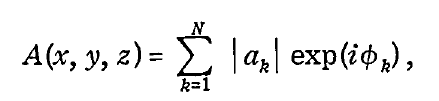# Real and imaginary part of SLC product

Hello,
I am new in radar and I have a naive doubt. As explained in

Moreira, Alberto, et al. “A tutorial on synthetic aperture radar.” IEEE Geoscience and remote sensing magazine, (2013)

“The received echo signal data form a two-dimensional data matrix of complex samples, where each complex sample is given by its real and imaginary part, thus representing an amplitude and phase value.”

But I also read that in SNAP, to derive the amplitude and the phase from the i,q layers, one should apply these equations:
The amplitude = sqrt(i^2 + q^2)
The phase = arctan (i / q)
(What does Bands specify in SNAP toolbox?)

So i and q are not just amplitude and phase? In Sentinel -1 SLC there is already a Virtual intensity band. How is it derived?
Thank youas phase and amplitude have different units, they are expressed by two complex numbers, i and q. Together they allow to derive both amplitude and phase by these formulas.
Amplitude squared gives intensity. If you move your cursor over the intensity band in the Table of Contents (or open its properties) you can see its equation.

i and q, as well as phase and amplitude, are two complementary ways to describe a complex number.

Complex numbers on the unit circle.

I wanted to ask this question here
Speckle filtering with Lee filters
but I have found this post more relevant since we discuss complex number.

I have found this formula frequently in literature(Goodman 1976). Apparently the phase must be expressed in complex values instead of simply be put in radians, or degrees.
in (Engdahl) said that the phase and amplitudes of the elementary scatters that are of size below the resolution cell are not observable and only their vector sum Ae^iφk can be observed. But I still don’t understand why the complex number is used in this equation.

Those sub-questions may help me understand this better if answered,

What is the difference between phase difference from elementary scatterers and the phase difference emerged from ground changes between two SAR acquisitions?

What if the phase difference that caused speckle effect was expressed in Radians or angles instead of complex number values to solve the sum of the contributions

It does not matter if one expresses a vector with angle (phase) and length, or with i and q, it is only a matter of which convention has been chosen. i and q was chosen for SNAP as that is the convention of most SLC products.

edit: also in the formula that you posted above the phase φ_k is in radians and is not complex.

1 Like

This may be a basic question but I have been searching and studying math, wave physics for weeks before I ask here but I couldn’t understand the full idea. How did you deduce the equation for the vector sum of elementary scatterer’s phasor amplitudes?

Have you derived it from the common wave equation of a harmonic sinusoidal wave equation?

f(x,t) = A sin (kx- 𝜔t+ θ )
where f(x,t) is the wave amplitude at time t and horizontal position x, and x is the position of the wave in space and k is the wavenumber, 𝜔 is the angular frequency, t is the time variable and θ is the phase of the wave.

Yes in complex notation, Euler’s Theorem explains what e^iφ_k is in sin() and cos() terms, see:

Euler’s Theorem - formula (2.3.2)

This Q&A explains well the use of complex numbers in electromagnetics:

https://physics.stackexchange.com/questions/566605/electromagnetic-waves-complex-numbers